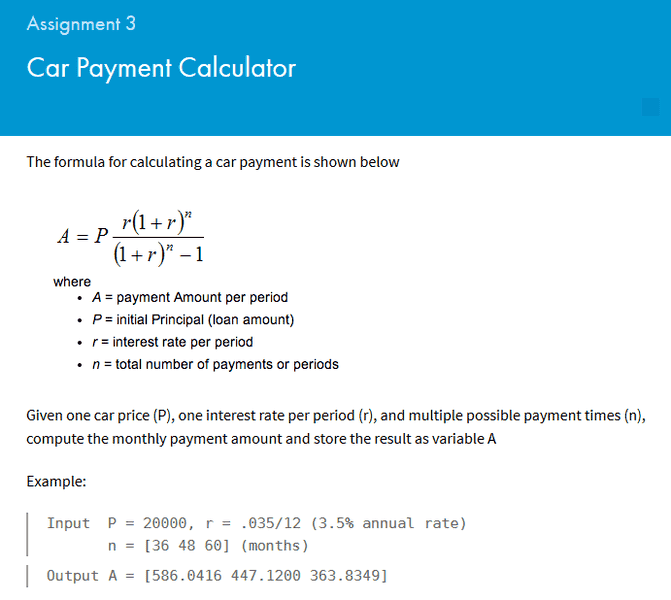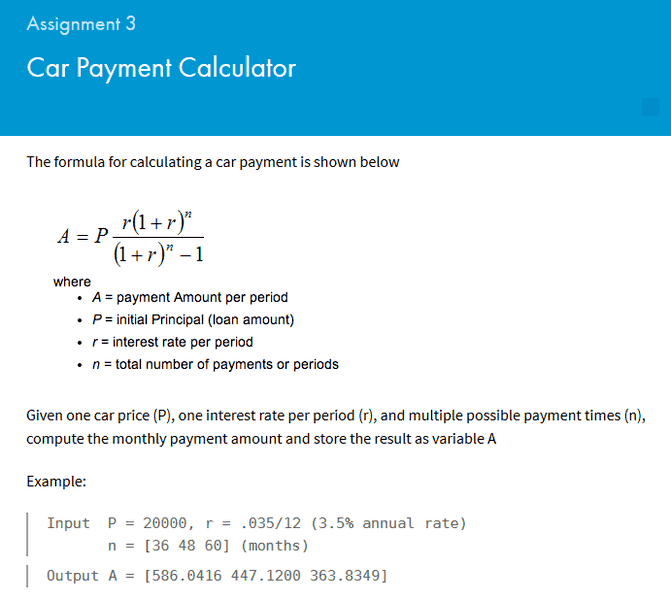# MATLAB: input arrays into function

• Feodalherren
In summary, the conversation discusses how to write code for scalars using variables P, r, and n. The code provided gives a value for A that is different from the expected value. The solution involves setting n as a vector and adding a period before operators that should be done as scalar operations.

## Homework Statement## The Attempt at a Solution

Okay, so first off I'm just trying to write the code for scalars:
--------------------------------------
clc ; clear

P = 2000
r = .035/12
n = 36

F = (1 + r).^n

A = (r*P*F)/(F-1)
---------------------------------------

This gives me A = 58.6042
which obviously isn't the same as the 586.0416 that they get in the example.

Feodalherren said:

## Homework Statement## The Attempt at a Solution

Okay, so first off I'm just trying to write the code for scalars:
--------------------------------------
clc ; clear

P = 2000
r = .035/12
n = 36

F = (1 + r).^n

A = (r*P*F)/(F-1)
---------------------------------------

This gives me A = 58.6042
which obviously isn't the same as the 586.0416 that they get in the example.
Check the value you're using for the loan amount P.

Ah, haha. Always something like that. Now for the actual problem that I was having; how do I make it possible for n to be an array and A to display an array of answers?

Maybe something like this:
Code:
clc ; clear
P = 20000
r = .035/12
n = [36 48 60]
A = zeros[1, 3]  ; Initialize A to [ 0 0 0]
F = zeros[1, 3] ; Initialize F to [ 0 0 0]

F = (1 + r) .^ n

A = (r*P*F)/(F-1)
I don't have Matlab, so I can't guarantee this will work

•Feodalherren
The code that you have for scalars is actually already pretty close to what you need. Instead of having n be a scalar, you are going to want to set up n to be a vector [36 48 60].

From there, all you need to do in the code, is anytime that you are wanting it to do scalar multiplication or division instead of vector multiplication or division, you will need to put a period before it to force MatLab to do the operation item by item.

F = (1 + r).^n (you already have your .^n here before your exponential, so you have that right).

This will provide you F as a vector for each of the associate values for n. From there you will just need to take the second part of your code and add a period before any of the operators that you want MatLab to do as a scalar operation (In your case, any operator that is using your new vector F).

•Feodalherren
I solved it
------------------------
F = (1 + r).^n

A = (r*P.*F)./(F-1)
--------------------------
Thanks guys.